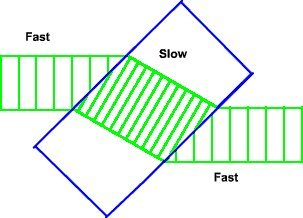<< I don't yet understand why when light passes through a medium, the wavelength changes. It seems to me that the frequency would change. In my mind I relate frequency to speed or velocity. I relate to what the "wavelength" is—i.e., a wavelength of 389 nm IS violet light. >>

The color of light is not primarily dependent on the wavelength of the light, as most people probably think. A wavelength of 389 nm is violet light in a vacuum, where the index of refraction is 1.000. However, in a medium where the index of refraction is greater than 1.000, the wavelength of violet light is not necessarily exactly 389 nm.

The color mainly is dependent upon the light's frequency, which is the number of waves of the light that pass a given point in one second. If the color of light was dependent on wavelength, its color would change when it entered a medium with a different index of refraction. But it doesn't. For instance, if you look at an object in air, and then jump into a swimming pool with the object and look at it under water, the color of the object will remain the same.

<< I understand that light "slows down" as it passes though a medium (other than a vacum or air), and to me that means the frequency must change. >>

Actually, no. Two things change when light goes from one medium to another: the speed of the light and the wavelength of the light. But the frequency of the series of the light waves, passing a given point per second, does not change; thus the color does not change.

Let's say laser light of a certain color (for instance, green) is aimed at an aquarium full of water. The light enters one side, passes through the water, and emerges from the other side. If you are at the top of the aquarium looking in, you will see that the beam of light is the same color outside of the aquarium, as well as inside of the glass/water. The reason for this is because of the following important formula:

speed of the light = frequency x wavelength

As the light enters the glass and water, it slows down. At the same time, the wavelength of the light also decreases. But the frequency (and, therefore, the color) remains the same, because the same number of waves are passing the same point in space per second both inside the glass/water and in the air outside. Here is a graphic depiction of this:As you can see, the wavelength of the green light shortens in the water, and so does the speed of the light. But the frequency remains the same; therefore, so does the color.

Ted
www.tedmontgomery.com